# 将一维时间序列转化成二维图片

• 数学先验知识；
• Gram Matrix 为何可以为单变量的时间序列构建一个好的二维表示；
• Gram Matrix 点积为何不能表示 CNN 的数据；
• 为 CNN 准备好 Gram Matrix 结构的操作是什么；

• 用于 GAF 计算的 numpy 工具；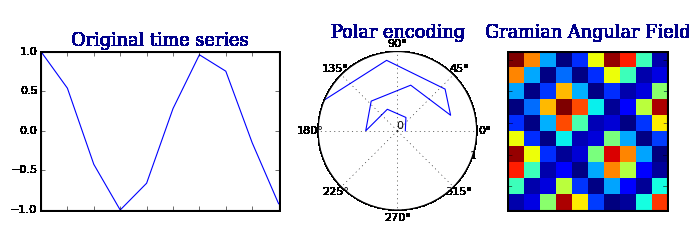### 1. 数学先验知识

GAF 的数学方法与内积与相应的 Gram 矩阵有很深的联系。

#### 1.1. 点积（Dot product）

$\langle u,v\rangle=u_1 \cdot v_1+u_2 \cdot v_2$

$\langle u,v\rangle=\|u\|\cdot \|v\|\cdot\cos{(\theta)}$

$\langle u,v\rangle=cos{(\theta)}$

$\langle u,v\rangle=\sum_{i=1}^{n}u_i\cdot v_i$

#### 1.2. Gram 矩阵（Gram Matrix）

$G=\begin{pmatrix} \langle u_1,v_1\rangle & \langle u_1,v_2\rangle & \cdots & \langle u_1,v_n\rangle \\ \langle u_2,v_1\rangle & \langle u_2,v_2\rangle & \cdots & \langle u_2,v_n\rangle \\ \vdots & \vdots & \ddots & \vdots \\ \langle u_n,v_1\rangle & \langle u_n,v_2\rangle & \cdots & \langle u_n,v_n\rangle \\ \end{pmatrix}$

$G=\begin{pmatrix} \cos{(\phi_{1,1})} & \cos{(\phi_{1,2})} & \cdots & \cos{(\phi_{1,n})} \\ \cos{(\phi_{2,1})} & \cos{(\phi_{2,2})} & \cdots & \cos{(\phi_{2,n})} \\ \vdots & \vdots & \ddots & \vdots \\ \cos{(\phi_{n,1})} & \cos{(\phi_{n,2})} & \cdots & \cos{(\phi_{n,n})} \\ \end{pmatrix}$

Gram 矩阵保留了时间依赖性。由于时间随着位置从左上角到右下角的移动而增加，所以时间维度被编码到矩阵的几何结构中。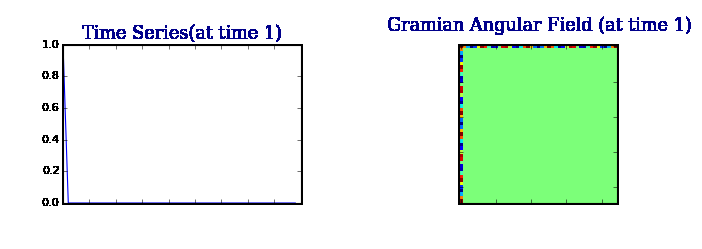：单变量时间序列在某种程度上无法解释数据的共现和潜在状态；我们的目标应该是找到替代的和更丰富的表示。

### 2. 实现方式

#### 2.1. 缩放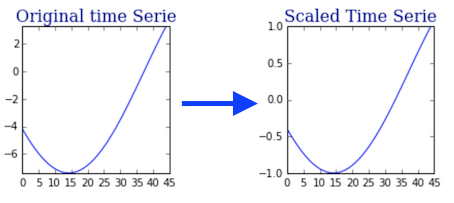$\langle\cdot,\cdot\rangle:[-1,1]\times[-1,1]\rightarrow[-1,1] \\ (x,y)\rightarrow x\cdot y$

#### 2.2. 噪声图片

$G=\begin{pmatrix} x_1\cdot x_1 & x_1\cdot x_2 & \cdots & x_1\cdot x_n \\ x_2\cdot x_1 & x_2\cdot x_2 & \cdots & x_2\cdot x_n \\ \vdots & \vdots & \ddots & \vdots \\ x_n\cdot x_1 & x_n\cdot x_2 & \cdots & x_n\cdot x_n \\ \end{pmatrix}$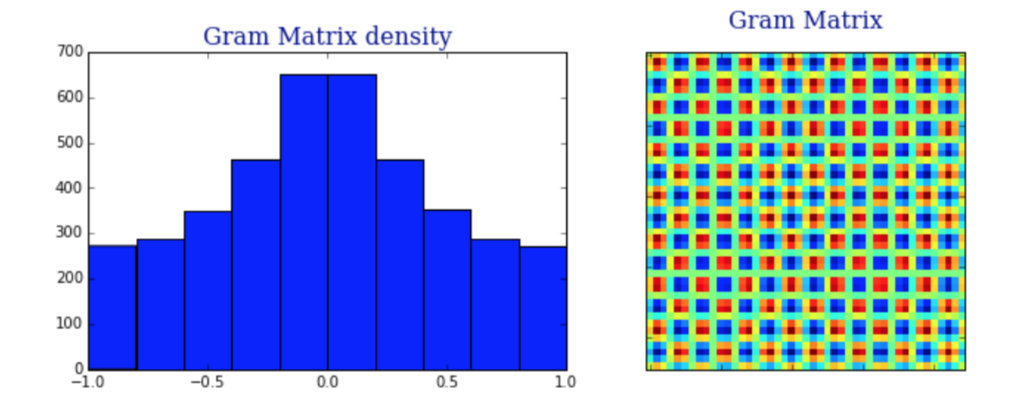1. 输出似乎遵循以 0 为中心的高斯分布。

2. 得到的图片是有噪声的。

#### 2.3. 非稀疏性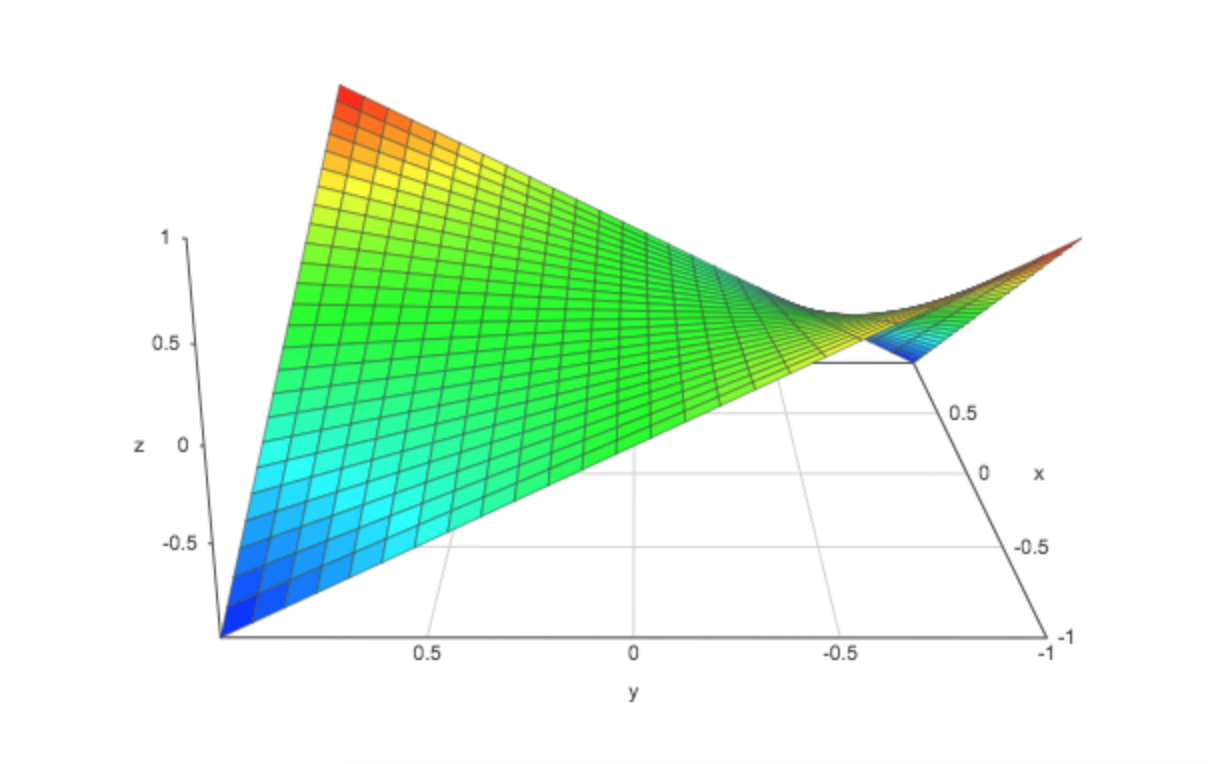### 3. 开始编码

#### 3.1. 缩放序列• 角度是用 $arccos(x)$ 计算的，值在 $[0,\pi]$ 之间。
• 首先计算半径变量，我们把区间 [0,1] 分成 $N$ 等份。因此，我们得到 $N+1$ 个分隔点 $\{0,\cdots,1\}$ 。然后我们丢弃 0，并连续地将这些点与时间序列关联起来。

$\begin{cases} \phi_i=arccos(x_i) \\ r_i=\frac{i}{N} \end{cases}$

1. 整个编码是双射的（作为双射函数的组合）。
2. 它通过 $r$ 坐标保持时间依赖性。这个优点很有用。

### 4. 时间序列的内积

#### 4.1. 为什么不是极坐标编码值的内积呢？

• 两个截然不同的观察结果之间的内积将偏向于最近的一个（因为范数随时间增加）；
• 当计算观测值与自身的内积时，得到的范数也是有偏差的。

#### 4.2. 使用角度

$x\oplus y=\cos{(\theta_1+\theta_2)}$

$G=\begin{pmatrix} \cos{(\phi_1+\phi_1)} & \cos{(\phi_1+\phi_2)} & \cdots & \cos{(\phi_1+\phi_n)} \\ \cos{(\phi_2+\phi_1)} & \cos{(\phi_2+\phi_2)} & \cdots & \cos{(\phi_2+\phi_n)} \\ \vdots & \vdots & \ddots & \vdots \\ \cos{(\phi_n+\phi_1)} & \cos{(\phi_n+\phi_2)} & \cdots & \cos{(\phi_n+\phi_n)} \\ \end{pmatrix}$

1. 对角线由缩放后的时间序列的原始值构成（我们将根据深度神经网络学习到的高层特征来近似重构时间序列）；
2. 时间相关性是通过时间间隔 $k$ 的方向叠加，用相对相关性来解释的。

#### 4.3. 稀疏表示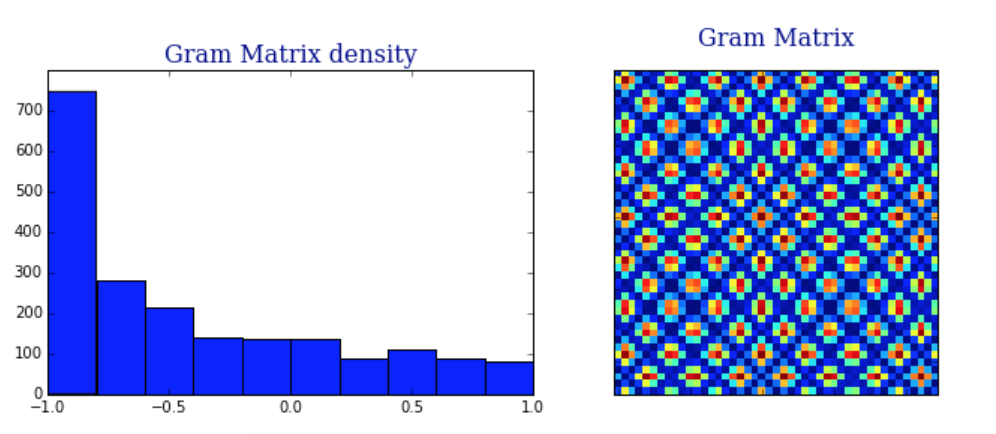$\begin{matrix} \cos(\theta_1+\theta_2) & = & cos(arccos(x)+arccos(y)) \\ & = & cos(acrccos(x))\cdot cos(arccos(y))-sin(arccos(x))\cdot sin(arccos(y)) \\ & = & x\cdot y+\sqrt{1-x^2}\cdot \sqrt{1-y^2} \\ & = & \langle x,y \rangle-\sqrt{1-x^2}\cdot \sqrt{1-y^2} \end{matrix}$

$x\oplus y=x\cdot y-\rangle-\sqrt{1-x^2}\cdot \sqrt{1-y^2}$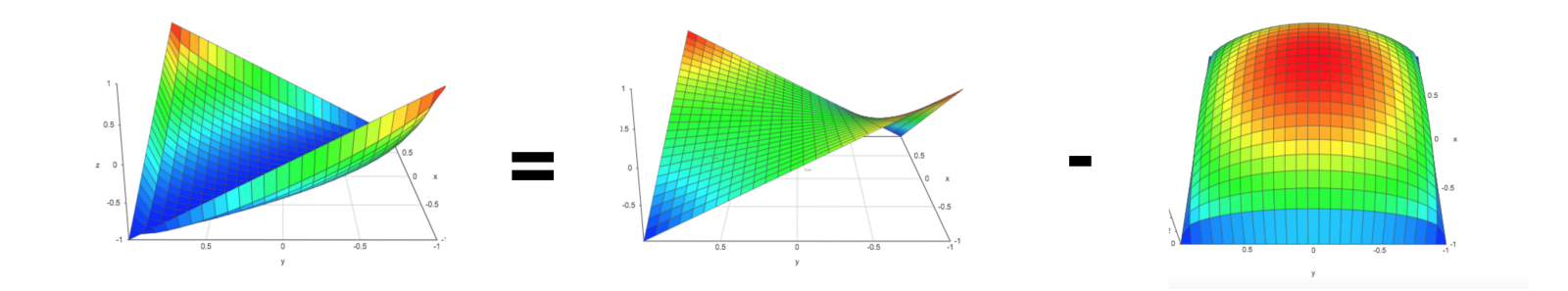• 惩罚将平均输出移向 -1；
• $x$$y$ 越接近0，惩罚越大。主要的原因是，这些点点更接近高斯噪声；
• 对于 $x=y$：会转换为 -1；
• 输出很容易与高斯噪声区分开。

• 主对角线,然而,生成的 GAM 很大是由于 $n\mapsto n^2$，而原始时间序列的长度为 n。作者建议通过使用分段聚合近似（Piecewise Aggregation Approximation）减少大小。
• 这个操作不是真正意义上的内积。

### 5. 代码

Github 上可以找到将单变量时间序列转换为图像的 numpy 实现。

05-044566

#### Python：使用 pyts 把一维时间序列转换成二维图片03-139976

#### 深度学习进行时间序列模式识别

02-26451

#### matlab+PYTHON 时频分析1D信号转换为2D时频图像

03-111万+

#### 一维卷积的意义和二维卷积（图像处理）的简单理解

11-251120

#### 将时间序列转换为监督学习数据

03-15829

#### cnn实现一维震动信号故障诊断

12-30737

#### GO 注释文件 GAF 详解

12-273304

#### 时间序列--可视化的几种方式

11-27263

#### 一维序列转二维坐标©️2020 CSDN 皮肤主题: 像素格子 设计师: CSDN官方博客点击重新获取扫码支付1.余额是钱包充值的虚拟货币，按照1:1的比例进行支付金额的抵扣。
2.余额无法直接购买下载，可以购买VIP、C币套餐、付费专栏及课程。余额充值